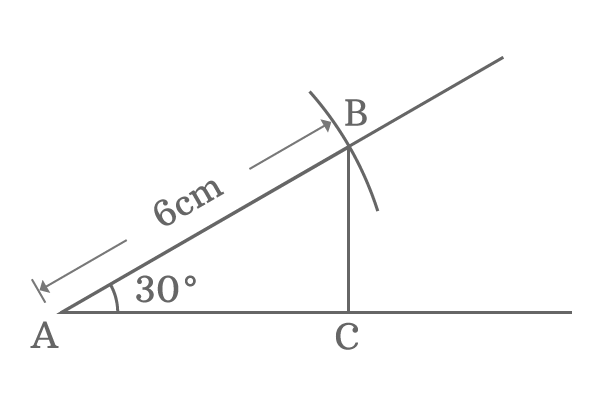# Sine value

## Definition

The value that calculated from the ratio of length of opposite side to length of hypotenuse at the respective angle of the right angled triangle is called sine value or value of sine of an angle.The value of sine of an angle is evaluated by comparing the length of the opposite side with length of hypotenuse by the ratio at that angle. It is useful to understand the length of the opposite side is how many times to length of the hypotenuse at the respective angle of the right angled triangle.

The value of sine of an angle depends on neither length of opposite side nor length of hypotenuse but depends on ratio between them and angle of the right angled triangle only. Trigonometric ratio sine has a unique value for every angle of the right angled triangle.

### How to find sine value

The value of sine for every angle is calculated by the geometric approach and it can be understood here from an example.1. Take scale and draw a horizontal line. The left side point of the horizontal line is called as point $A$.
2. Take protractor, coincide point $A$ with centre of the protractor and also coincide horizontal line with right side base line of the protractor. Mark a point at $30$ degrees angle.
3. Take scale once again, draw a long straight line from point $A$ through $30$ degrees angle marked point.
4. Use compass and set the length to $6$ centimeters, and then draw an arc on $30$ degrees straight line from point $A$. Call the intersecting point of straight line and arc as point $B$.
5. Take set square and draw a perpendicular line to horizontal line from point $B$. The perpendicular line from point $B$ intersects the horizontal line at point $C$.

Thus, a right angled triangle, known as $\Delta BAC$, is constructed geometrically with an angle of $30^\circ$ and $6$ centimetres length. The $6$ centimetres long straight line becomes hypotenuse of this right angled triangle but length of the opposite side is unknown. So, take scale and measure the length of the opposite side and it will be exactly $3$ centimeters.

Now calculate sine of angle $30^\circ$

$$\sin 30^\circ = \frac{Length \, of \, Opposite \, side}{Length \, of \, Hypotenuse}$$

$$\implies \sin 30^\circ = \frac{BC}{AB}$$

$$\implies \sin 30^\circ = \frac{3}{6}$$

$$\implies \sin 30^\circ = \frac{1}{2} = 0.5$$

According to right angled triangle $\Delta BAC$, the value of sine of angle $30^\circ$ is derived as $0.5$ from the ratio of length of the opposite side to length of the hypotenuse.

Construct another right angled triangle with different length but same angle.$\Delta EDF$ is constructed with angle of $30^\circ$ and $7$ centimetres length line by the same procedure. Measure the length of the opposite side, it will be $3.5$ centimetres exactly.

Calculate sine of angle $30^\circ$

$$\implies \sin 30^\circ = \frac{EF}{DE}$$

$$\implies \sin 30^\circ = \frac{3.5}{7}$$

$$\implies \sin 30^\circ = \frac{1}{2} = 0.5$$

According to the right angled triangle $\Delta EDF$, the value of sine of angle $30^\circ$ is also derived as $0.5$ from the ratio of length of the opposite side to length of the hypotenuse.

Construct another right angled triangle with different length but same angle.

Construct another right angled triangle one last time with different length but same angle.$\Delta HGI$ is also constructed with angle $30^\circ$ and $10$ centimetres line by using same process. Measure the length of opposite side. It will be $5$ centimetres exactly. Then calculate sine of $30^\circ$

$$\implies \sin 30^\circ = \frac{HI}{GH}$$

$$\implies \sin 30^\circ = \frac{5}{10}$$

$$\implies \sin 30^\circ = \frac{1}{2} = 0.5$$

According to right angled triangle $\Delta HGI$, the value of sine of angle $30^\circ$ is also derived as $0.5$ from the ratio of length of the opposite side to length of the hypotenuse.

#### Result

Geometrically, we have constructed three right angled triangles $\Delta BAC$, $\Delta EDF$ and $\Delta HGI$ with same angle but different lengths, and also obtained from them that

1. The lengths of opposite side and hypotenuse of $\Delta BAC$ are $3$ and $6$ centimeters.
2. The lengths of opposite side and hypotenuse of $\Delta EDF$ are $3.5$ and $7$ centimetres.
3. The lengths of opposite side and hypotenuse of $\Delta HGI$ are $5$ and $10$ centimeters.

All three triangles are right angled triangles. Lengths of opposite sides of three triangle are different and also lengths of hypotenuse of all three triangles are also different but the ratios of lengths of opposite sides to hypotenuses are same because the angle is same.

It is proved that the value of sine of angle only depends on angle and ratio of length of the opposite side to length of the hypotenuse of the right angled triangle. So, you can construct a right angled triangle for any angle with any length by using same geometric procedure to find the value of sine of any angle in trigonometry.

Latest Math Topics
Latest Math Problems
Email subscription
Math Doubts is a best place to learn mathematics and from basics to advanced scientific level for students, teachers and researchers. Know more
Follow us on Social Media
###### Math Problems

Learn how to solve easy to difficult mathematics problems of all topics in various methods with step by step process and also maths questions for practising.

Learn more